Dynamic Programming

Dynamic Programming

1 × 2 × 3 × ⋯ × N。整數1到N的連乘積。N階乘。N!。

N!源自(N-1)!，如此就遞迴分割問題了。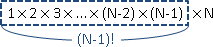UVa 623 568 10220 10323

(recurrence)

Dynamic Programming
= Divide-and-Conquer Method + Memoization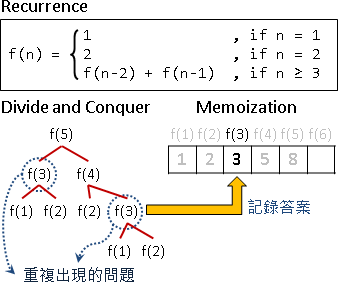```1. 把原問題遞迴分割成許多更小的問題。（recurrence）
1-1. 子問題與原問題的求解方式皆類似。（optimal sub-structure）
1-2. 子問題會一而再、再而三的出現。（overlapping sub-problems）
2. 設計計算過程：
2-1. 確認每個問題需要哪些子問題來計算答案。（recurrence）
2-2. 確認總共有哪些問題。（state space）
2-3. 把問題一一對應到表格。（lookup table）
2-4. 決定問題的計算順序。（computational sequence）
2-5. 確認初始值、計算範圍。（initial states / boundary）
3. 實作，主要有兩種方式：
3-1. Top-down
3-2. Bottom-up
```

1. recurrence

【註：recursion和recurrence，中文都翻譯為「遞迴」，然而兩者意義大不相同，讀者切莫混淆。】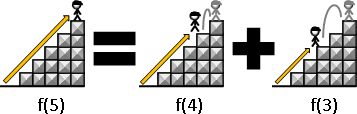「爬到五階」的踏法數目，就是總合「爬到四階」與「爬到三階」的踏法數目。寫成數學式子是「f(5) = f(4) + f(3)」，其中「f(‧)」表示「爬到某階之踏法數目」。

「爬到兩階」與「爬到一階」無法再分割、沒有子問題，直接得到「f(2) = 2」、「f(1) = 1」。

```f(n) =
{ 1                , if n = 1
{ 2                , if n = 2
{ f(n-1) + f(n-2)  , if n >= 3 and n <= 5
```2-1. recurrence

```f(n) =
{ 1                , if n = 1
{ 2                , if n = 2
{ f(n-1) + f(n-2)  , if n >= 3
```2-2. state space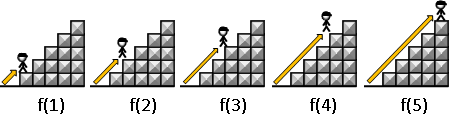2-3. lookup table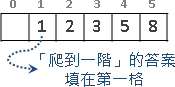2-4. computational sequence2-5. initial states / boundary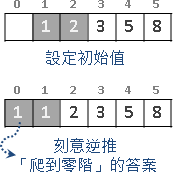```f(n) =
{ 0                , if n < 0               [Exterior]
{ 1                , if n = 0               [Initial State]
{ 1                , if n = 1               [Initial State]
{ f(n-1) + f(n-2)  , if n >= 2 and n <= 5   [Computation]
{ 0                , if n > 5               [Exterior]
```

UVa 11069

3. 實作

```1. Top-down
2. Bottom-up
```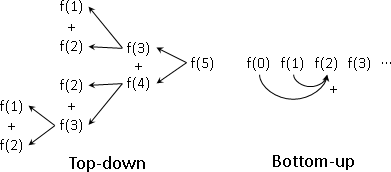3-1. Top-down

UVa 10285 10446 10520

3-2. Bottom-up

UVa 495 900 10334

(counting / optimization)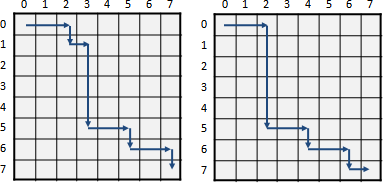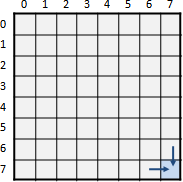「從上走來」是一個規模更小的問題，「從左走來」是一個規模更小的問題，答案是兩者相加。UVa 10599 825 926

```f(i, j) =
{ 0                      , if i < 0 or j < 0     [Exterior]
{ 1                      , if i = 0 or j = 0     [Initial States]
{ f(i-1, j) + f(i, j-1)  , if i > 0 and i < 8    [Computation]
{                          and j > 0 and j < 8
{ 0                      , if i >= 8 or j >= 8   [Exterior]

f(i, j)：從格子 (0, 0) 走到格子 (i, j) 的走法數目。
```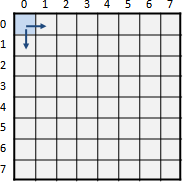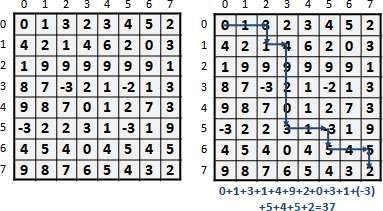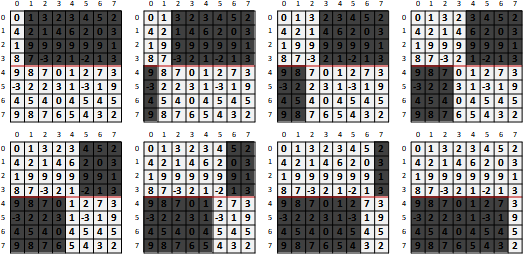(state / DAG)

state / DAG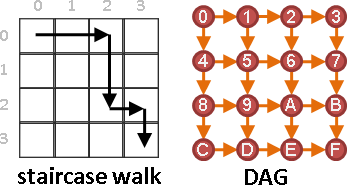```f(n) =
{ f(n-1) + f(n-2)  , if n > 2
{ 2                , if n = 2
{ 1                , if n = 1
``````f(n) =
{ f(n-1) + 1 + f(n-1)  , if n > 1
{ 1                    , if n = 1
``````f(h, w) =
{ f(h-1, w) + f(h, w-1)  , if h > 1 and w > 1
{ 1                      , if h = 1 or w = 1
```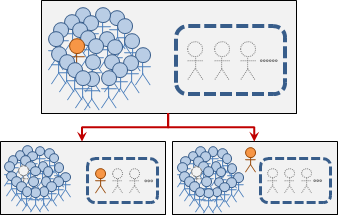```f(n, m) =
{ f(n-1, m-1) + f(n-1, m)  , if n > 1 and m > 1 and m < n
{ 1                        , if n = 1 or n = m
```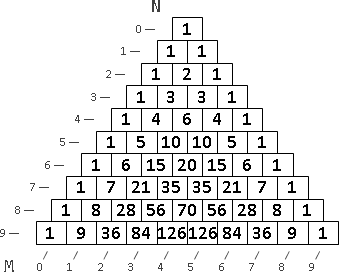🡲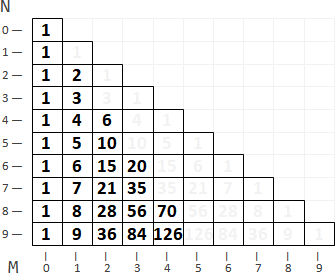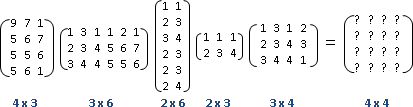```f(i, k) =  min  { f(i, j) + f(j+1, k) + r[i] ⋅ c[j] ⋅ c[k] }
j=i⋯k-1

f(i, k)：從第i個矩陣乘到第k個矩陣，最少的相乘次數。
r[i]：第i個矩陣的row數目。
c[i]：第i個矩陣的column數目。
```

```Matrix Chain Multiplication
Optimal Binary Search Tree
Hu–Tucker Compression
Minimum Weight Triangulation of Convex Polygon
Cocke–Younger–Kasami Algorithm
```

UVa 348 442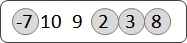```f(j) = max ( 1, max { f(i) + ( a[i] < a[j] ? 1 : -∞ ) } )
i=0⋯j-1

f(j)：前j個數字可以挑出的數字數量，而且第j個數字被挑出。
a[j]：第j個數字。
```

```Longest Increasing Subsequence
Longest Common Subsequence
Longest Palindromic Subsequence
```

UVa 10900(bitset)

bitset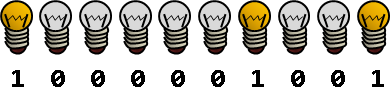bitset是一個二進位數字，每一個bit分別代表每一件東西，1代表開啟，0代表關閉。例如現在有十個燈泡，編號設定為零到九，其中第零個、第三個、第九個燈泡是亮的，剩下來的燈泡是暗的。我們用一個10 bit的二進位數字1000001001，表示這十個燈泡的亮暗狀態。

UVa 10952Maximum Matching」有多項式時間演算法，可是很難實作；動態規劃雖然慢了些，是指數時間演算法，但是容易實作。移除匹配成一對的點，就得到遞迴公式。

```f(S+{i}+{j}) = max { f(S) + adj(i, j) }
i,j∉S
```
```f(S) = max { f(S-{i}-{j}) + adj(i, j) }
i,j∈S
```

UVa 10888 10911 11439 10296 11156Hamilton Path」尚無多項式時間演算法。直覺的解法是backtracking，窮舉所有點的各種排列方式，一種排列方式當作一條路徑，判斷是不是Hamilton Path。

```f(S+{j}, j) = or { f(S, i) and adj(i, j) }   where j∉S
i∈S
```
```f(S, j) = or { f(S-{j}, i) and adj(i, j) }   where j∈S
i∈S
i≠j
```

UVa 216 10068 10496 10818 10937 10944 10605 10890 265

UVa 11741

UVa 10572 10531

Dynamic Programming Speedup

Dynamic Programming Speedup

(stack / deque)

```F[n] = min/max { F[i] ⋅ W[i] + C[i] }
i=0⋯n-1
```

F[n]是未知數，F[i] W[i] C[i]是已知數。計算到F[n]時，F[i]早已計算完畢，因此F[i]是已知數。

stack

```          for finding maximum,
keep monotone increasing  ---->
┌──────┬──────┬──────┬─────────┬───────╯
stack │ M │ M │ M │   ...   │ M
└──────┴──────┴──────┴─────────┴───────╮
^^^^^^^ extract maximum
clean tails until monotonicity
then push new M[i] into tail
```

M[i] = F[i] ⋅ W[i] + C[i]塞入尾端。塞入前，先彈出尾端數值們，使得M[i]塞入尾端之後，stack嚴格遞增。最大值從尾端取得。

deque

```           for finding minimum,
keep monotone increasing  ---->
╰──────┬──────┬──────┬─────────┬───────╯
deque   M │ M │ M │   ...   │ M
╭──────┴──────┴──────┴─────────┴───────╮
^^^^^^                         ^^^^^^^
extract minimum      clean tails until monotonicity
then push new M[i] into tail
```

M[i] = F[i] ⋅ W[i] + C[i]塞入尾端。塞入前，先彈出尾端數值們，使得M[i]塞入尾端之後，deque嚴格遞增。塞入後，再彈出頭端數值們，使得deque符合滑動視窗範圍。最小值從頭端取得。

(envelope / convex hull)

```F[n] = min/max { F[i] ⋅ W[n] + C[i] }
i=0⋯n-1
```

W[i]換成W[n]。處理方式大幅改變。

envelopeconvex hull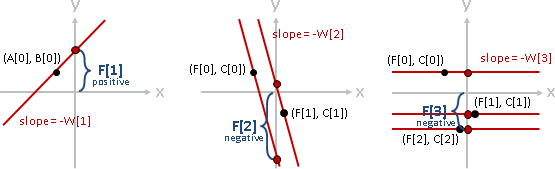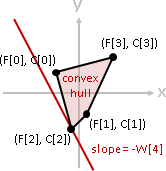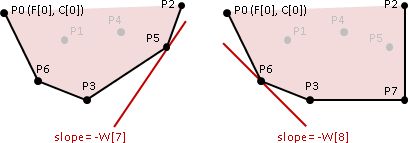UVa 12524

(unimodal function)

```F[n] = min/max { M[i] }
i=0⋯n-1

M[i] is monotone/unimodal
```

```F[n] =  min  { max(F[i], G[i]) + 5 }
i=0⋯n-1

(1) F[i] is increasing
(2) G[i] is decreasing
(=>) max(F[i], G[i]) + 5 is unimodal
```

F遞增、G遞減，則max(F[i], G[i]) + 5是單峰函數。不過這個例子有點蠢，山谷恰好永遠不動。```F[n] =  min  { F[i] + G[i] }
i=0⋯n-1

(1) F[i] is convex
(2) G[i] is convex
(=>) F[i] + G[i] is convex (also unimodal)
```

F G皆是凸函數，則F[i] + G[i]是凸函數，即是單峰函數。不過這個例子也有點蠢，山谷恰好永遠不動。```F[n] = 1 +  min  { max(i-1, F[n-i]) }
i=1⋯n
```
```F[n] = 1 +  min  { max(i, F[n-1-i]) }   調整索引值
i=0⋯n-1
```

i遞增，F[n-1-i]遞減，因此max(i, F[n-1-i])是單峰函數，而且山谷持續往右移動，可以使用掃描線解決。另外也有數學公式解：

UVa 10934 882

(Monge matrix)

```F[n] = min/max { M[i][n] }
i=0⋯n-1

M[i][n] is upper-triangular monotone/totally monotone/Monge
```

```F[n] = min/max { F[i] ⋅ W[i] + C[i][n] }
i=0⋯n-1

(1) C[i][n] is upper-triangular Monge
(=>) M[i][n] = F[i] ⋅ W[i] + C[i][n] is upper-triangular Monge
```

Monge matrix橫條加上同一數，仍是Monge matrix。藉由這個數學性質，我們得以處理這種形式的recurrence。

convex totally monotone matrix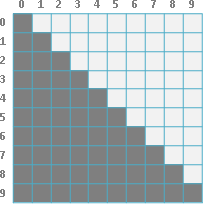concave totally monotone matrix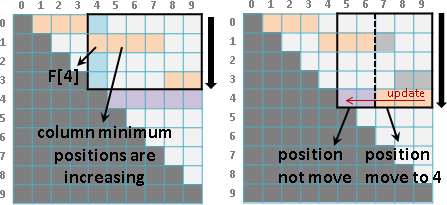(interval)

Knuth–Yao speedup

```F[i,j] =  min  { F[i,k] + F[k+1,j] + C[i,j] }
k=i⋯j-1

(1) C[i,j] is Monge*:  C[a,b] + C[a+1,b+1] ≤ C[a,b+1] + C[a+1,b]
(2) C[i,j] is sorted:  C[a,b] ≤ C[a,b+1] and C[a,b] ≤ C[a-1,b]
(C[a,b] ≤ C[c,d] when [a,b] ⊆ [c,d])
(=>) F[i,j] is Monge*
(=>) P[i,j] is sorted: P[a,b] ≤ P[a,b+1] and P[a,b] ≤ P[a+1,b]
(P[a,b-1] ≤ P[a,b] ≤ P[a+1,b])

*non-negative upper-trianglar concave Monge
```
```(1) C[i,j] is Monge:   ↖ + ↘ ≤ ↗ + ↙       (concave)
(2) C[i,j] is sorted:  ← ≤ → and ↓ ≤ ↑     (toward ↗)
(←↙↓ ≤ →↗↑)
(=>) F[i,j] is Monge
(=>) P[i,j] is sorted: ← ≤ → and ↑ ≤ ↓     (toward ↘)
(↖ ≤ ↗ ≤ ↘)
```

N筆資料，欲建立成一棵「Binary Search Tree」。並且預測了每筆資料的搜尋次數。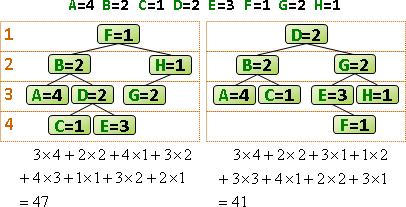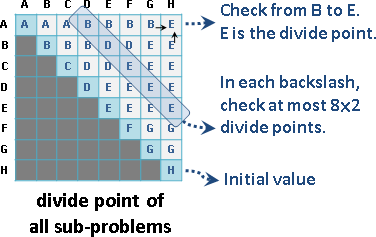UVa 10304 10003 12057 12809

Hu–Shing speedup

```F[i,j] =  min  { F[i,k] + F[k+1,j] + C[i,j,k] }
k=i⋯j-1
```

【尚無正式名稱】

```F[i][j] =  min  { F[i-1][k-1] + C[k,j] }
k=1⋯j

(1) C[i,j] is Monge: C[a,b] + C[a+1,b+1] ≤ C[a,b+1] + C[a+1,b]
(=>) P[i][j] is row-sorted: P[i,j] ≤ P[i,j+1]

(2) C(|i-j|) is convex
(=>) F[i][j] is row-sorted
(=>) F[i-1][k-1] + C[k,j] is unimodal
```

```[envelope/convex hull] [unimodal function]
using geometric techniques to improve dynamic programming algorithms for the economic lot-sizing problem and extensions

[Monge matrix]
dynamic programming with convexity, concavity and sparsity
an almost linear time algorithm for generalized matrix searching

[Knuth–Yao speedup]
optimum binary search trees
efficient dynamic programming using quadrangle inequalities

[Hu–Shing speedup]
computation of matrix chain products, part I, part II
revisiting “computation of matrix chain products”
```